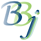# SQL — HEX() Function

## Syntax

HEX(str,start,length)

 Parameter Description str The hexadecimal argument to be subdivided. start A number that represents the beginning position in the expanded hex string. length A number that represents the length in hex digits from the starting position in the expanded hex string. A hex digit is equal to four bits or half a byte.

## Description

The HEX() function decodes a decimal-encoded hexadecimal field. This means that when a HTA() function of the field is taken, the result can be construed as a decimal string. For example, the number 4876 will be stored in a hex field as \$4876\$. Binary fields can be thought of as integers of the specified length.

## Example

Select HEX(myfield.binary_data,1,2) from myfile